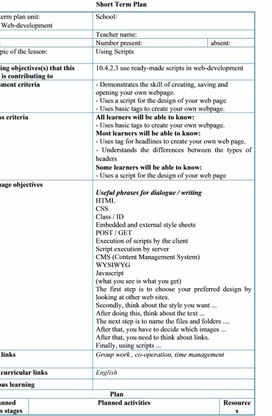1_Computer science grade 10Using Scripts_lesson_plan1_2 variant
Оценка 4.9

# 1_Computer science grade 10Using Scripts_lesson_plan1_2 variant

Оценка 4.9
docx
09.05.20201_Computer science grade 10Using Scripts_lesson_plan1_2 variant.docx

## Short Term Plan

Appendix 1

Javascript calendar

<script language = "javascript">

function montharr (m0, m1, m2, m3, m4, m5, m6, m7, m8, m9, m10, m11) {

this  = m0;

this  = m1;

this  = m2;

this  = m3;

this  = m4;

this  = m5;

this  = m6;

this  = m7;

this  = m8;

this  = m9;

this  = m10;

this  = m11;

}

function calendar () {

var monthNames = "JanFevMarAprMayIJunIjulAugSenOctNewDec";

var today = new Date ();

var thisDay;

var monthDays = new montharr (31, 28, 31, 30, 31, 30, 31, 31, 30.31, 30, 31);

year = today.getYear ();

if (year <2000)

year = year + 1900;

thisDay = today.getDate ();

if (((year% 4 == 0) && (year% 100! = 0)) || (year% 400 == 0))

monthDays  = 29;

nDays = monthDays [today.getMonth ()];

firstDay = today;

firstDay.setDate (1);

testMe = firstDay.getDate ();

if (testMe == 2)

firstDay.setDate (0);

startDay = firstDay.getDay ();

document.writeln ("<div align = 'center'>");

document.write ("<table border = '1'>");

document.write ("<tr> <th colspan = '7'>");

document.write (monthNames.substring (today.getMonth () * 3 + 3, (today.getMonth () + 1) * 3 + 3));

document.write (".");

document.write (year);

document.write ("<tr> <th> Sun <th> Mon <th> W <th> Wed <th> Th <th> Fri <th> Sat");

document.write ("<tr>");

column = 0;

for (i = 0; i <startDay; i ++) {

document.write ("<td width = '30 '>");

column ++;

}

for (i = 1; i <= nDays; i ++) {

document.write ("<td width = 30>");

if (i == thisDay)

document.write ("<span style = 'color: red;'>")

document.write (i);

if (i == thisDay)

document.write ("</ span>")

column ++;

if (column == 7) {

document.write ("<tr>");

column = 0;

}

}

document.write ("</ table>");

document.writeln ("</ div>");

}

</ script>

HTML code (insert between the <body> and </ body> tags):

<script language = 'javascript'>

calendar ();

</ script>

(source: https://myrusakov.ru/kalendar-javascript.html)

2.1 How much time did you spend on the page?

<script language = "javascript">

startdate = new Date ();

clockStart = startdate.getTime ();

function initStopwatch () {

var thisTime = new Date ();

return (thisTime.getTime () - clockStart) / 1000;

}

function getSecs () {

var tSecs = Math.round (initStopwatch ());

var iSecs = tSecs% 60;

var iMins = Math.round ((tSecs-30) / 60);

var sSecs = "" + ((iSecs> 9)? iSecs: "0" + iSecs);

var sMins = "" + ((iMins> 9)? iMins: "0" + iMins);

document.getElementById ("timer-counter"). innerHTML = sMins + ":" + sSecs;

setTimeout ('getSecs ()', 1000);

}

</ script>

HTML code (insert between <body onLoad = "getSecs ()"> and </ body> tags:

<span id = 'timer-counter' style = 'color: red; font-size: 150%; font-weight: bold;'> </ span>

minutes / seconds.

(source: https://myrusakov.ru/kalendar-javascript.html)

Скачано с www.znanio.ru

Скачать файл